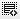## How to calculate MPV?0
If you invested Rs. 10 lakhs (1 million) in a project and expect to get a steady cash flow of Rs. 1 lakhs (100,000) every year over the next 5 years. What is the NPV of this project assuming prevailing interest rates of 15%?

Rs. 615,067 - Incorrect

Rs.-6,64,784

Rs. 500

Rs. 600,000

plese expaln me the calculation in detail sir please0

Yashodha. This question focuses on calculating NPV of an investment in the project.
Cash inflow is Rs.10 lakhs
Cash outflow is Rs. 1 lakh every year for 5 years
Discount Rate is 15%
if you lay out these numbers is excel as -10,00,000, 1,00,000, 1,00,000, 1,00,000, 1,00,000, 1,00,000, and 15%. Then use the excel formula =NPV(rate,.......) you should be able to get the answer.

Click on thisicon to add code snippet.